• 它可以绘制各种图形，下面就学习了下Python中的matplotlib模块，如何绘制三维图形。示例代码一：# coding=utf-8 import numpy as np import matplotlib.pyplot as plt import mpl_toolkits.mplot3d a,b = np.mgrid[....
Python matplotlib模块是扩展的MATLAB的一个绘图工具库。它可以绘制各种图形，下面就学习了下Python中的matplotlib模块，如何绘制三维图形。示例代码一：# coding=utf-8
import numpy as np
import matplotlib.pyplot as plt
import mpl_toolkits.mplot3d
a,b = np.mgrid[-2:2:20j,-2:2:20j]
#测试数据
c=a*np.exp(-a**2-b**2)
#三维图形
ax = plt.subplot(111, projection='3d')
ax.set_title('www.linuxidc.com - matplotlib Code Demo');
ax.plot_surface(a,b,c,rstride=2, cstride=1, cmap=plt.cm.Spectral)
#设置坐标轴标签
ax.set_xlabel('A')
ax.set_ylabel('B')
ax.set_zlabel('C')
plt.show()运行结果：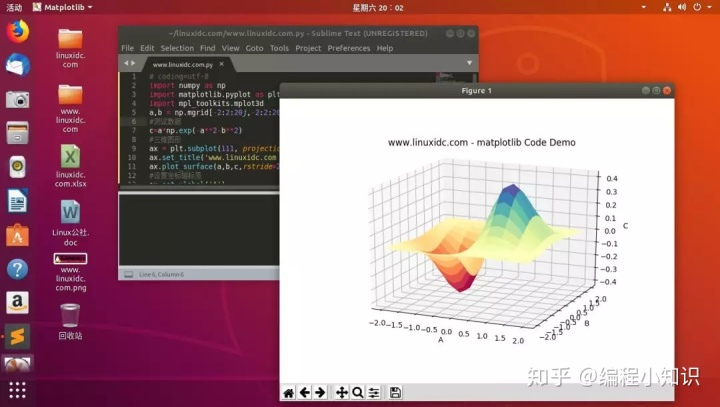示例代码二：# coding=utf-8
import pylab as pl
import numpy as np
import mpl_toolkits.mplot3d
rho, theta = np.mgrid[0:1:40j,0:2*np.pi:40j]
c = rho**2
a = rho*np.cos(theta)
b = rho*np.sin(theta)
ax = pl.subplot(111, projection='3d')
ax.set_title('www.linuxidc.com - matplotlib Code Demo');
#ax.plot_surface(a,b,c)
ax.plot_surface(a,b,c,rstride=2, cstride=1)
#设置坐标轴标签
ax.set_xlabel('A')
ax.set_ylabel('B')
ax.set_zlabel('C')
pl.show()运行结果：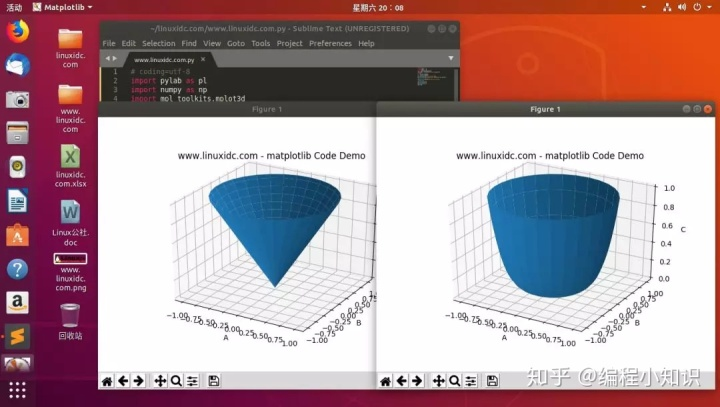如果您在学习编程的过程中遇到难题，欢迎关注微信公众号【筑梦前端】，大家一起交流解决！
展开全文• 他可以绘制各种图形，可是最近最的一个小程序，得到一些三维的数据点图，就学习了下python中的matplotlib模块，如何绘制三维图形。 初学者，可能对这些第三方库安装有一定的小问题，对于一些安装第三方库经验较少的...
python matplotlib模块，是扩展的MATLAB的一个绘图工具库。他可以绘制各种图形，可是最近最的一个小程序，得到一些三维的数据点图，就学习了下python中的matplotlib模块，如何绘制三维图形。

首先提醒注意，以下两个函数的区别：

ax.plot_surface(X, Y, Z, rstride=1, cstride=1, cmap='rainbow') #绘面

和

ax.scatter(x[1000:4000],y[1000:4000],z[1000:4000],c='r') #绘点

1、绘制3D曲面图

# -*- coding: utf-8 -*-
"""
Created on Thu Sep 24 16:17:13 2015

@author: Eddy_zheng
"""

from matplotlib import pyplot as plt
import numpy as np
from mpl_toolkits.mplot3d import Axes3D

fig = plt.figure()
ax = Axes3D(fig)
X = np.arange(-4, 4, 0.25)
Y = np.arange(-4, 4, 0.25)
X, Y = np.meshgrid(X, Y)
R = np.sqrt(X**2 + Y**2)
Z = np.sin(R)

# 具体函数方法可用 help(function) 查看，如：help(ax.plot_surface)
ax.plot_surface(X, Y, Z, rstride=1, cstride=1, cmap='rainbow')

plt.show()

效果: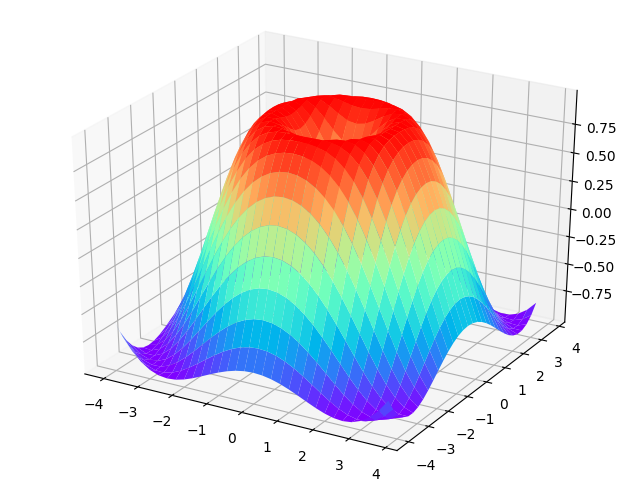2、绘制三维散点图

# -*- coding: utf-8 -*-
"""
Created on Thu Sep 24 16:37:21 2015

@author: Eddy_zheng
"""
import numpy as np
import matplotlib.pyplot as plt
from mpl_toolkits.mplot3d import Axes3D

data = np.random.randint(0, 255, size=[40, 40, 40])

x, y, z = data, data, data
ax = plt.subplot(111, projection='3d')  # 创建一个三维的绘图工程
#  将数据点分成三部分画，在颜色上有区分度
ax.scatter(x[:10], y[:10], z[:10], c='y')  # 绘制数据点
ax.scatter(x[10:20], y[10:20], z[10:20], c='r')
ax.scatter(x[30:40], y[30:40], z[30:40], c='g')

ax.set_zlabel('Z')  # 坐标轴
ax.set_ylabel('Y')
ax.set_xlabel('X')
plt.show()

效果：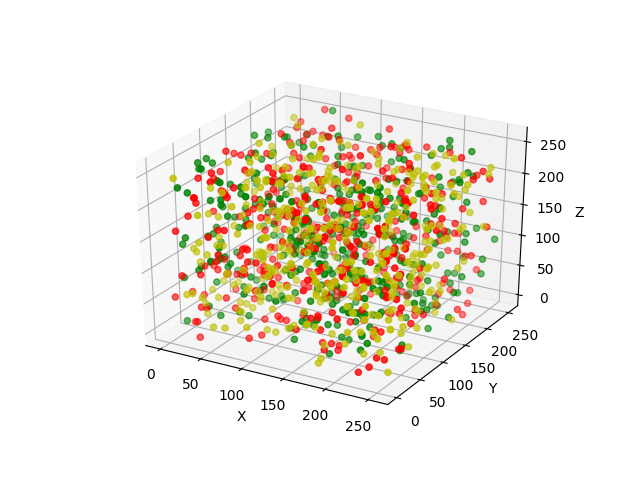上面就是学习区分了下两个函数，当时还被小困惑了下，希望对大家有所帮助。其实里面还有好多参数设置，比如说改变颜色，包括绘制点图的点的形状等都是可以改变的，有需要的大家可以自己看看这个函数，学习下(help(对应的function))。
展开全文python matplotlib 数据
• 他可以绘制各种图形，可是最近最的一个小程序，得到一些三维的数据点图，就学习了下python中的matplotlib模块，如何绘制三维图形。初学者，可能对这些第三方库安装有一定的小问题，对于一些安装第三方库经验较少的...
分类： 计算机视觉python matplotlib模块，是扩展的MATLAB的一个绘图工具库。他可以绘制各种图形，可是最近最的一个小程序，得到一些三维的数据点图，就学习了下python中的matplotlib模块，如何绘制三维图形。初学者，可能对这些第三方库安装有一定的小问题，对于一些安装第三方库经验较少的朋友，建议使用 Anaconda ，集成了很多第三库，基本满足大家的需求，下载地址，对应选择python 2.7 或是 3.5 的就可以了（PS：后面的demo是python2.7）：https://www.continuum.io/downloads#windows首先提醒注意，以下两个函数的区别：ax.plot_surface(X, Y, Z, rstride=1, cstride=1, cmap='rainbow') #绘面和ax.scatter(x[1000:4000],y[1000:4000],z[1000:4000],c='r') #绘点1、绘制3D曲面图# -*- coding: utf-8 -*-"""Created on Thu Sep 24 16:17:13 2015@author: Eddy_zheng"""from matplotlib import pyplot as pltimport numpy as npfrom mpl_toolkits.mplot3d import Axes3Dfig = plt.figure()ax = Axes3D(fig)X = np.arange(-4, 4, 0.25)Y = np.arange(-4, 4, 0.25)X, Y = np.meshgrid(X, Y)R = np.sqrt(X**2 + Y**2)Z = np.sin(R)# 具体函数方法可用 help(function) 查看，如：help(ax.plot_surface)ax.plot_surface(X, Y, Z, rstride=1, cstride=1, cmap='rainbow')plt.show()效果展示：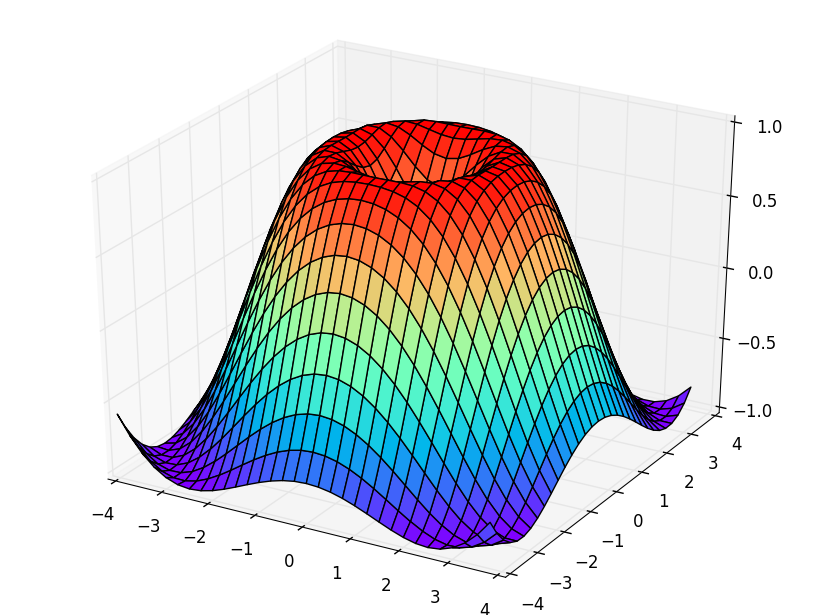2、绘制三维的散点图（通常用于表述一些数据点分布）效果：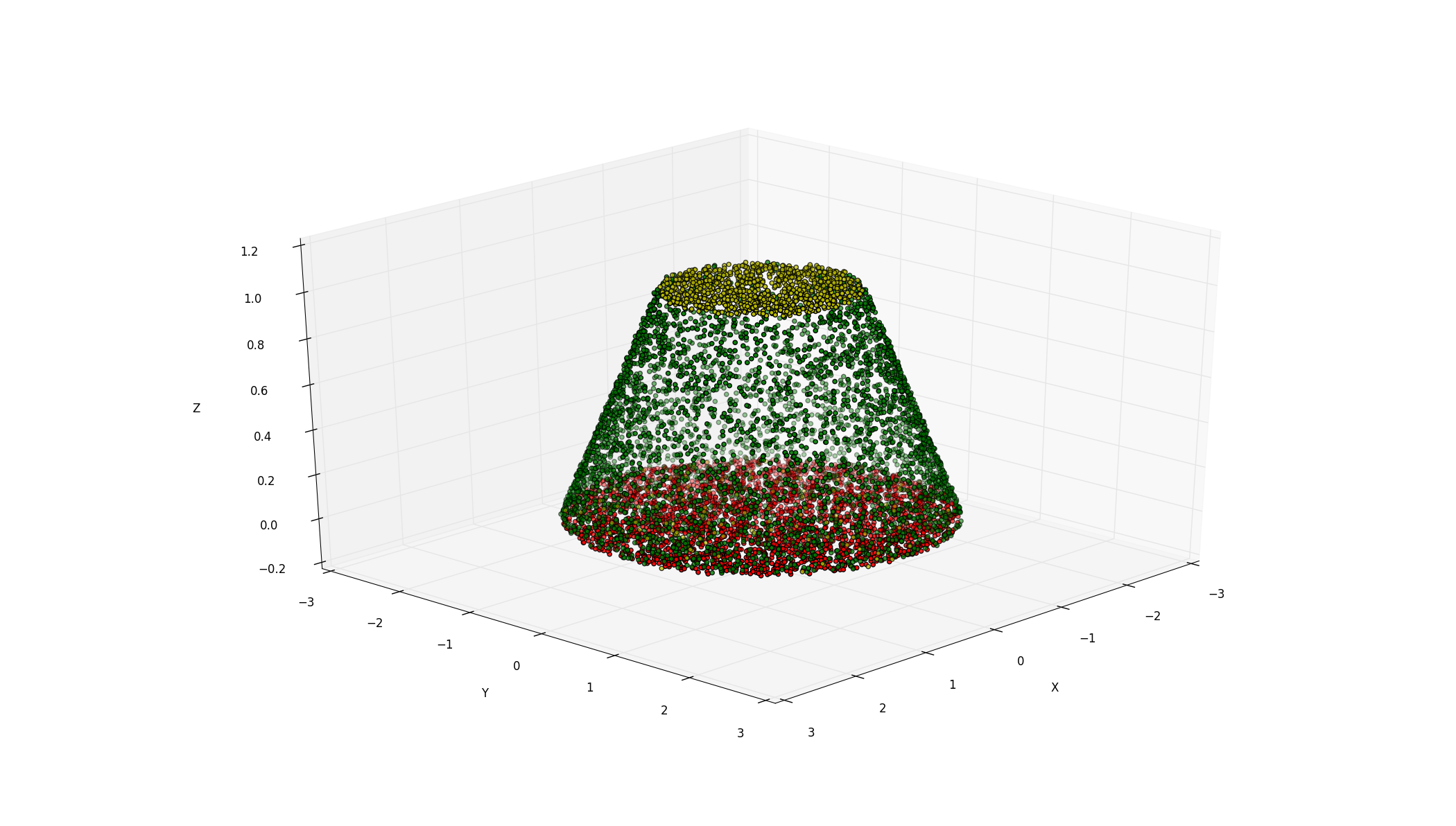# -*- coding: utf-8 -*-"""Created on Thu Sep 24 16:37:21 2015@author: Eddy_zheng"""import scipy.io as siofrom mpl_toolkits.mplot3d import Axes3Dimport matplotlib.pyplot as pltmat1 = '4a.mat' #这是存放数据点的文件，需要它才可以画出来。上面有下载地址data = sio.loadmat(mat1)m = data['data']x,y,z = m,m,max=plt.subplot(111,projection='3d') #创建一个三维的绘图工程#将数据点分成三部分画，在颜色上有区分度ax.scatter(x[:1000],y[:1000],z[:1000],c='y') #绘制数据点ax.scatter(x[1000:4000],y[1000:4000],z[1000:4000],c='r')ax.scatter(x[4000:],y[4000:],z[4000:],c='g')ax.set_zlabel('Z') #坐标轴ax.set_ylabel('Y')ax.set_xlabel('X')plt.show()
展开全文• 它可以绘制各种图形，下面就学习了下Python中的matplotlib模块，如何绘制三维图形。 示例代码一： #coding=utf-8 importnumpyasnp importmatplotlib.pyplotasplt importmpl_toolkits.mplot3d a,b=np.m...
Python matplotlib模块是扩展的MATLAB的一个绘图工具库。它可以绘制各种图形，下面就学习了下Python中的matplotlib模块，如何绘制三维图形。

示例代码一：

# coding=utf-8
import numpy as np
import matplotlib.pyplot as plt
import mpl_toolkits.mplot3d
a,b = np.mgrid[-2:2:20j,-2:2:20j]
#测试数据
c=a*np.exp(-a**2-b**2)
#三维图形
ax = plt.subplot(111, projection='3d')
ax.set_title('www.linuxidc.com - matplotlib Code Demo');
ax.plot_surface(a,b,c,rstride=2, cstride=1, cmap=plt.cm.Spectral)
#设置坐标轴标签
ax.set_xlabel('A')
ax.set_ylabel('B')
ax.set_zlabel('C')
plt.show()

小编整理的一整套系统的python学习教程从最基础的的python脚本到web开发、爬虫、数据分析、数据可视化、机器学习的学习资料都有整理，送给每一位小伙伴, 有想学习python编程的，或是转行，或是大学生，还有工作中想提升自己能力的，正在学习的小伙伴欢迎加入学习。
加维信：Pythonsusu

运行结果：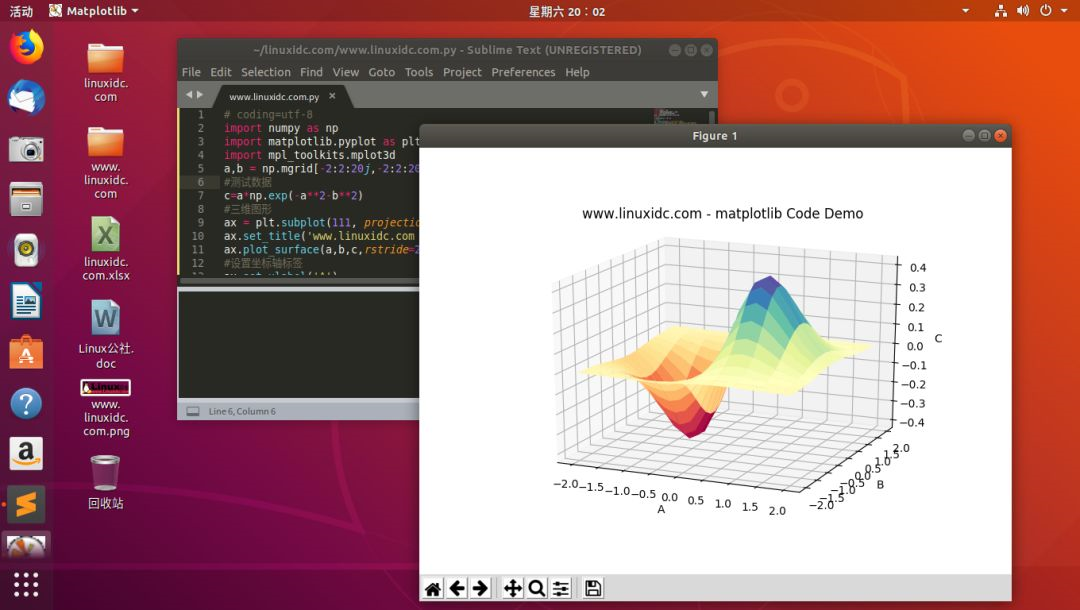示例代码二：

# coding=utf-8
import pylab as pl
import numpy as np
import mpl_toolkits.mplot3d
rho, theta = np.mgrid[0:1:40j,0:2*np.pi:40j]
c = rho**2
a = rho*np.cos(theta)
b = rho*np.sin(theta)
ax = pl.subplot(111, projection='3d')
ax.set_title('www.linuxidc.com - matplotlib Code Demo');
#ax.plot_surface(a,b,c)
ax.plot_surface(a,b,c,rstride=2, cstride=1)
#设置坐标轴标签
ax.set_xlabel('A')
ax.set_ylabel('B')
ax.set_zlabel('C')
pl.show()

运行结果：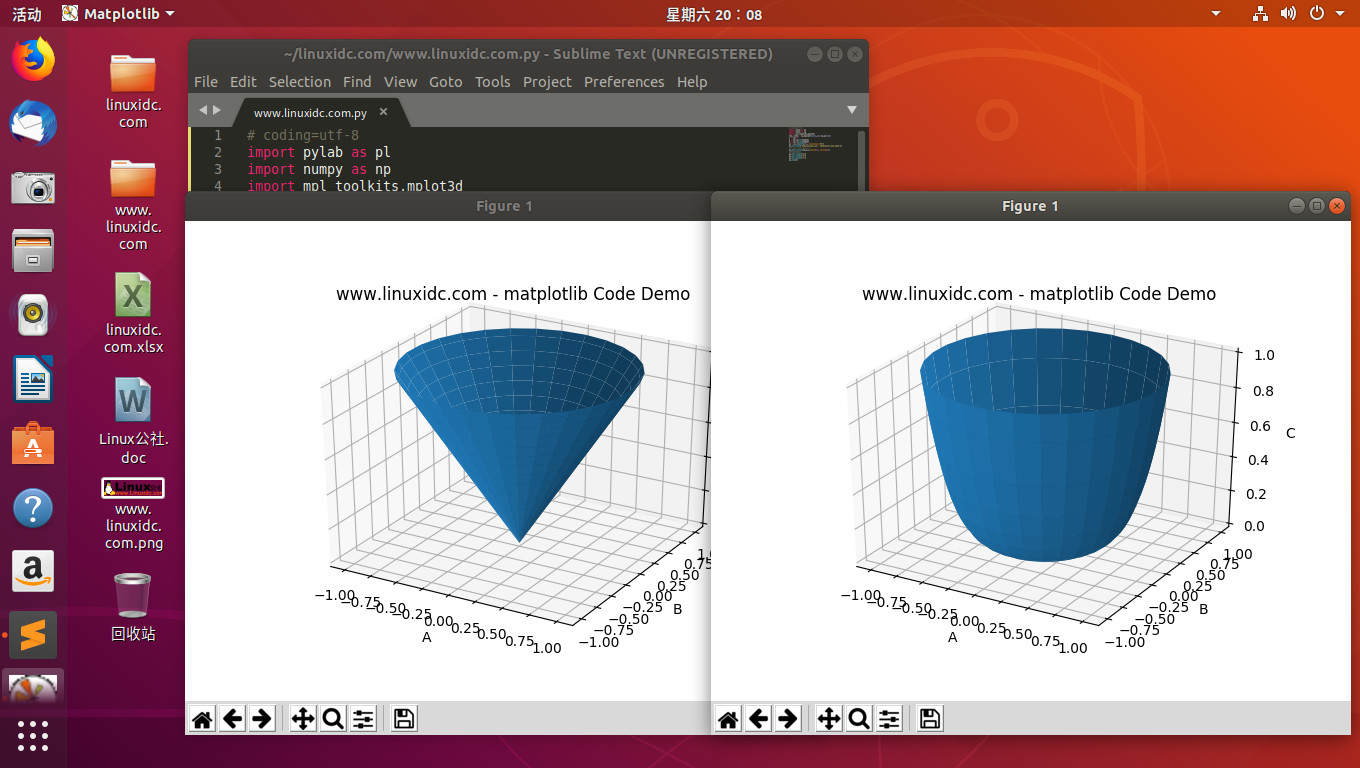展开全文• 它可以绘制各种图形，下面就学习了下Python中的matplotlib模块，如何绘制三维图形。示例代码一：# coding=utf-8import numpy as npimport matplotlib.pyplot as pltimport mpl_toolkits.mplot3da,b = np.mgrid[-2:2....
• 这个问题我以前回答过，使用的是matplotlib包绘制3D图形，包括3D散点图、3D折线图、3D柱状图、3D曲面图等，地址https://www.wukong.com/question/6582113875695501581/，这里我再介绍一种方式—pyecharts，echarts的...
• 三维图形一． 三维曲线plot3(x1,y1,z1,选项1,x2,y2,z2,选项2,…,xn,yn,zn,选项n)其中每一组x,y,z 组成一组曲线的坐标参数，选项的定义和plot 函数相同。当x,y,z 是同维向量时，则x,y,z 对应元素构成一条三维曲线。当...
• 它可以绘制各种图形，下面就学习了下Python中的matplotlib模块，如何绘制三维图形。示例代码一：# coding=utf-8import numpy as npimport matplotlib.pyplot as pltimport mpl_toolkits.mplot3da,b = np.mgrid[-2:2....
• 他能够绘制各类图形，但是最近最的一个小程序，获得一些三维的数据点图，就学习了下python中的matplotlib模块，如何绘制三维图形。小程序初学者，可能对这些第三方库安装有必定的小问题，对于一些安装第三方库经验较...
• 本项目展示了如何使用二维的绘图API (DrawLine)绘制三维图形的轮廓，开发语言为C#，项目环境为Visual Studio 2008。 三维旋转矩阵是投影操作中的关键，本项目展示了旋转矩阵的使用，并建立了一个简单的三维场景，...
• 我应该通过python读取这些数据，从而使用matplotlib生成一个3D图形。我看过很多pyton代码，但我不知道如何从数据中找出x、y和z轴。我知道这是一个模糊而蹩脚的问题，但我不熟悉这个问题，数学也很差。在在数据.txt在...
• 例如，有三维数组(int a)，现要将数据（以点的形式）在三维空间中显示出来，并且前5对数据显示为红色点，后5对数据显示为蓝色点。 请给出完整代码。 谢谢。c语言
• 从这篇文章开始，接下来会连载一系列的OpenGL相关博文，好好探讨...对于Android开发者来说，OpenGL就是用来绘制三维图形的技术手段，当然OpenGL并不仅限于展示静止的三维图形，也能用来播放运动着的三维动画。不管是三android OpenGL Render
• 在python中，我们常用matplotlib作图库来进行图形绘制，下面就是介绍python关于如何使用作图的步骤：工具/原料笔记本电脑python3.6方法/步骤1首先我们打开python软件，具体如图所示：2其次，我们要安装一些第方...
• 下面是一些如何将图像和图形图像数据显示为三维数据的示例。在第一个和第二个图显示原始BGR图像及其各个通道作为BGR，然后显示为LAB第三和第四张图显示了使用实验室图像的第一个通道作为三维数据的等高线图和曲面图...
• 如何在MFC中利用OpenGL实现三维图形绘制如何在MFC中利用OpenGL实现三维图形绘制如何在MFC中利用OpenGL实现三维图形绘制
• 写论文，从二维变三维空间，对三维工作空间分析，希望创建漂亮的工作空间示意图。二维区域着色已经在之前博文提到过了：Matlab不规则区域渐变色填充及注意事项。这里主要讲三维曲面着色。 方法 实现绘制曲面的函数有...
• 简要上一篇已经对图形的属性有过介绍，在此基础上来进行二维以及后续三维图形的操作(注：没接触过其他维度的操作，就不折腾相关的内容了)，将会更容易理解这些属性的用法，当然，全部的属性使用都来一遍，感觉就不太...
• 关于matlab如何绘图，包括（ppt资料，绘制二维曲线的最基本函数，绘制图形的辅助操作，绘制二维图形的其他函数，）对函数自适应采样的绘图函数，绘制三维曲线的最基本函数matlab
• 通常我们用 Python 绘制的都是二维平面图，但有时也需要绘制三维场景图，比如像下面这样的：这些图怎么做出来呢？今天就来分享下如何一步步绘制出三维矢量（SVG）图。八面体我们先以下面这个八面体为例。1 安装相关...
• 说明：本文中的数据是以矩阵...目录第一步：导入数据第二步：画图三维效果对比 第一步：导入数据 方式一： for tt = 1:1 filename = sprintf('测试数据\\Data%d.txt',tt);%生成文本名 fid_doa = fopen(filename...
• 3D图形在数据分析、数据建模、图形和图像处理等领域中都有着广泛的应用，下面将给大家介绍一下如何在Python中使用 matplotlib进行3D图形绘制，包括3D散点、3D表面、3D轮廓、3D直线（曲线）以及3D文字等的绘制。......